# Test Prep Plan - Take a practice test

Take this practice test to check your existing knowledge of the course material. We'll review your answers and create a Test Prep Plan for you based on your results.
How Test Prep Plans work
1
2Based on your results, we'll create a customized Test Prep Plan just for you!
3Study smarter
Study more effectively: skip concepts you already know and focus on what you still need to learn.

# The Derivative at a Point Chapter Exam

Exam Instructions:

Choose your answers to the questions and click 'Next' to see the next set of questions. You can skip questions if you would like and come back to them later with the yellow "Go To First Skipped Question" button. When you have completed the practice exam, a green submit button will appear. Click it to see your results. Good luck!

### Page 2

#### Question 6 6. What is the slope of the line normal to f(x) at x=1?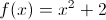#### Question 9 9. What is the rate of change at the point E in the graph below (for y in relation to x)?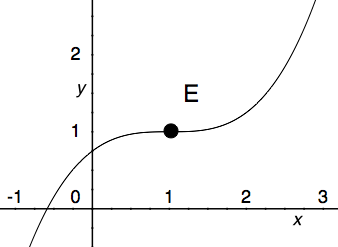### Page 3

#### Question 15 15. What is the slope of a line normal to f(x)?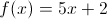### Page 4

#### Question 17 17. What is the slope of the tangent to r(s) at s = 0?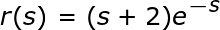#### Question 18 18. What is the rate of change at the point B in the graph below (for y in relation to x)? (In other words, what is the slope of the line from x = 2 to x = 3?)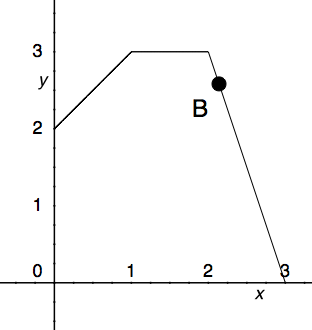#### Question 19 19. What is the equation of the line normal to f(x) at the point x=1?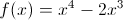### Page 5

#### Question 21 21. What is the rate of change at the point A in the graph below (for y in relation to x)?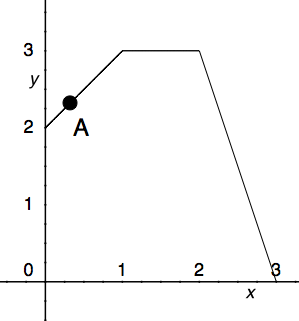#### Question 24 24. What is the rate of change at the point A in the graph below (for y in relation to x)?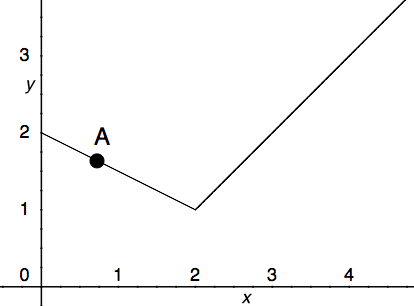#### Question 25 25. What is the slope of the line tangent to the curve given by the equation y = (x-1)²+2 at the point (1,2)?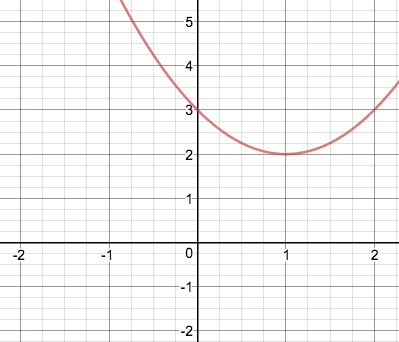### Page 6

#### Question 27 27. What is the equation of the line tangent to f(x) at the point x=0?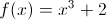#### Question 29 29. What is the rate of change at the point B in the graph below (for y in relation to x)?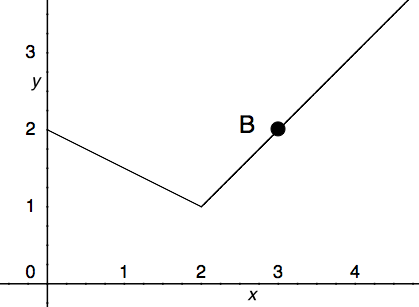#### The Derivative at a Point Chapter Exam Instructions

Choose your answers to the questions and click 'Next' to see the next set of questions. You can skip questions if you would like and come back to them later with the yellow "Go To First Skipped Question" button. When you have completed the practice exam, a green submit button will appear. Click it to see your results. Good luck!

Support